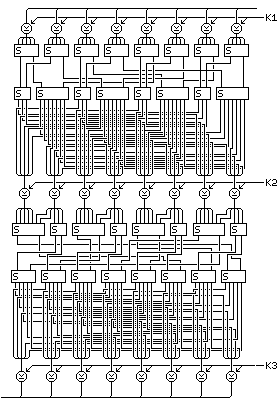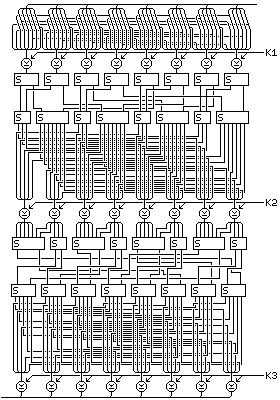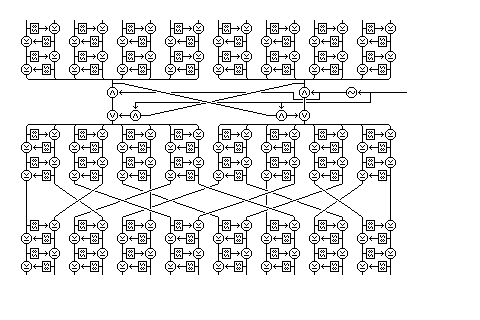[Next] [Up] [Previous] [Index]

# A Base Conversion Block Cipher and Other Concepts

### Block Cipher with Base Conversions

Another interesting idea for a block cipher happens to lead to one with an 80-bit block size, which has the advantage of being a multiple of five bits as well as eight, for use with characters in older systems using 5-level code.

Most block ciphers involve using S-boxes that operate on groups of bits, alternating with transpositions of individual bits, and XORs of subkey material. Some involve other binary operations.

But if one converts to other number bases from binary, one can shuffle around fractions of a bit, thus adding another kind of complexity to the design.

The fact that 33 is both 3 times 11 and one more than 32 is used, along with the fact that 9 is close to 8, and 121 is close to 128. Key-dependent S-boxes (since fixed ones would introduce bias, which could be exploited) operating in both directions are used, for example, both one with 33 entries consisting of all 32 5-bit combinations plus one duplicate, and one with 32 entries containing all but one of the 33 combinations of one 3-symbol and one 11-symbol.

The following are the steps that would comprise an f-function for a block cipher based on this construct:

1) XOR subkey K1 with the 40 bit input.

2) Take the bits of the input, 1 2 3 4 5 6 7 8 9 10 11 12 13 14 15 and so on, in groups of five, and using eight key-dependent S-boxes, turn each one into a 3-symbol and an 11-symbol, the 3-symbols being called a, b, c, d, e, f, g, and h respectively, and the 11-symbols being called S, T, U, V, W, X, Y, and Z.

3) Using four key-dependent S-boxes that produce three output bits from two 3-symbols, and another four key-dependent S-boxes that produce seven output bits from two 11-symbols, use the following symbol pairs as input:

```(a,b) (S,W) (c,d) (T,X) (e,f) (U,Y) (g,h) (V,Z)
```

4) The binary result of the above step is to recieve an additional bit permutation, as follows:

```11 22 33 14 25 36  7 18 29 40   21 32  3 24 35  6 17 28 39 10
31  2 13 34  5 16 27 38  9 20    1 12 23  4 15 26 37  8 19 30
```

5) Subkey K2 is XORed to the result.

6) Using key-dependent S-boxes that operate in the reverse direction, producing two 3-symbols from three bits (thus having eight entries with one combination omitted) and two 11-symbols from seven bits, produce

```S' T' a' b' U' V' c' d' W' X' e' f' Y' Z' g' h'
```

from the current result.

7) Group the symbols into the following pairs to produce, from eight key-dependent S-boxes, eight groups of five bits:

```(a',Z') (b',V') (c',Y') (d',U') (e',X') (f',T') (g',W') (h',S')
```

8) Apply the following bit permutation to the S-box outputs (numbers represent sources of bits, and are in the positions of result bits, as in the DES standard):

``` 6 17 23 29 40   11 22 28 34  5   16 27 33 39 10   21 32 38  4 15
26 37  3  9 20   31  2  8 14 25   36  7 13 19 30    1 12 18 24 35
```

9) XOR subkey K3 to the result.

and the following diagram illustrates this f-function:If an expansion permutation is desired as part of the f-function, to improve nonlinearity and to reduce the danger of bias, one logical place to put one would be before the XOR of the first subkey, making the first set of S-boxes, containing pairs of one 3-symbol and one 11-symbol as entries, 256 entries in size. This means that the first subkey increases in length, to become 64 bits long.

In generating these S-boxes in this form, it would make sense to first select eight different symbol pairs to omit in each of the eight permutations of 32 symbol pairs of which such a box should be composed.

An appropriate expansion permutation might have the form:

```40 23  6  1  2  3  4  5    5 28 11  6  7  8  9 10
10 33 16 11 12 13 14 15   15 38 21 16 17 18 19 20
20  3 26 21 22 23 24 25   25  8 31 26 27 28 29 30
30 13 36 31 32 33 34 35   35 18  1 36 37 38 39 40
```

and a revised diagram with the expansion permutation addedlooks like the above.

### Fractal Feistel

Another bright idea I had toyed with I discarded as excessively inefficient and probably insecure; but when the release of Skipjack indicated that the micro-Feistel rounds I used as the basis of this might actually be secure, I drew the following diagram to illustrate it:The basic idea behind this has been used before by others, for example in the MISTY block cipher developed by Mitsuru Matsui of Mitsubishi, and in the block cipher DEAL proposed by Richard Outerbridge as an AES candidate, that is: using a block cipher with Feistel rounds as the f-function for a larger block cipher with twice the block size. However, the diagram illustrates taking this to extremes.

The innermost function operates on a 16 bit block with four rounds, using a 256-byte lookup table as the f-function; this is the same as the "G permutation" in Skipjack.

That function is used as the f-function for four rounds within a block cipher acting on 32 bit blocks, which is, in turn, used for four rounds as the f-function of a block cipher operating on 64-bit blocks.

The diagram only includes one instance of this cipher - acting as the f-function for the actual block cipher, which operates on a 128-bit block.

Essentially, the Feistel round structure is replicated inside itself repeatedly, creating a block cipher with a fractal structure.

This design may have serious security flaws, but it is at least interesting to look at.

### Another Sketch

The following diagram:illustrates the rounds of a type of cipher that may well be secure even though it tries to be efficient and though it is limited to operations that are efficient on general-purpose computers.

Feistel rounds using a key-dependent S-box (called "S8" in the diagram, due to Quadibloc II) but no subkeys are combined with an ICE-style interchange between block halves and a fixed interchange of bytes designed to cause diffusion different from that provided by the cipher's other components to form four rounds, consisting of two batches of four mini-Feistel rounds and one ICE-style interchange, with three fixed byte interchanges between them.

[Next] [Up] [Previous] [Index]